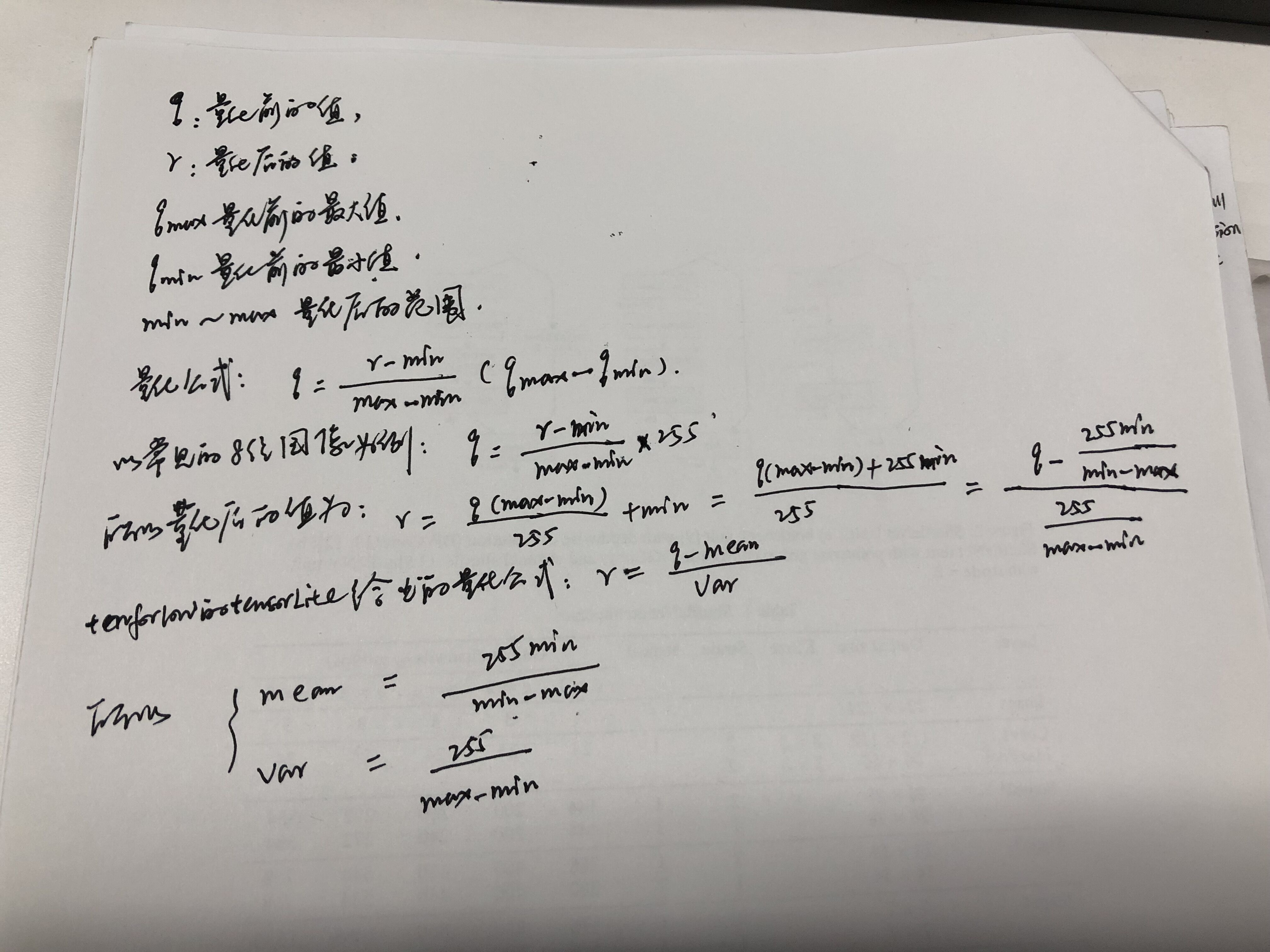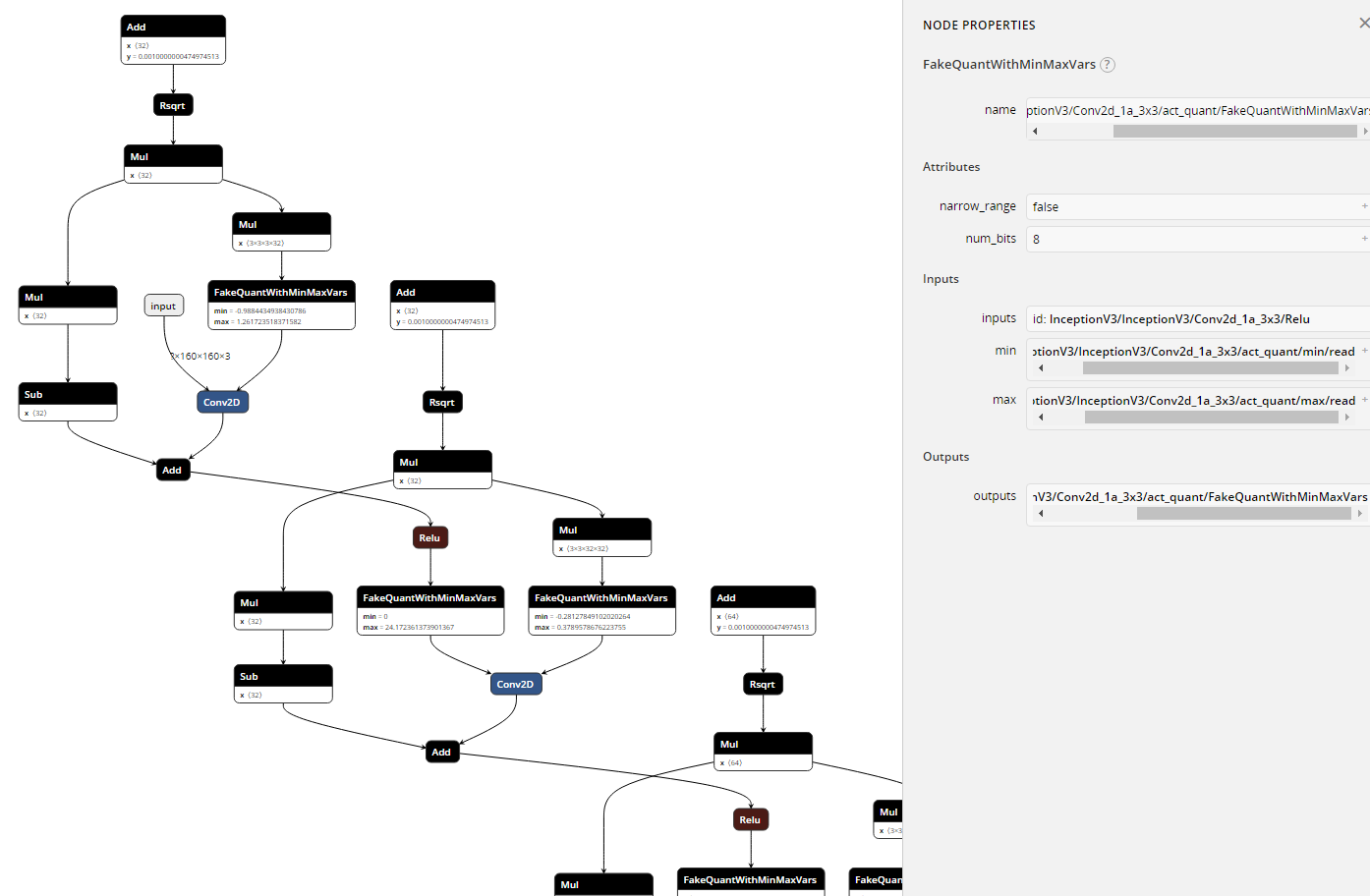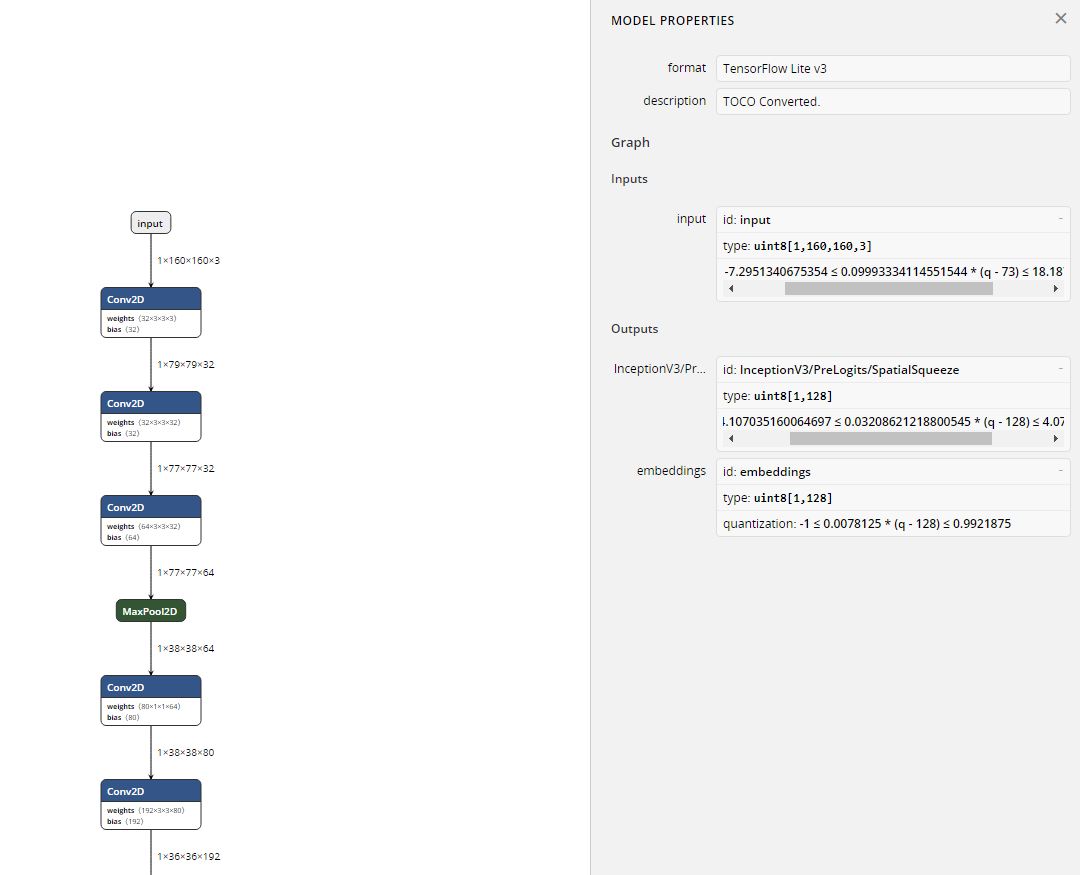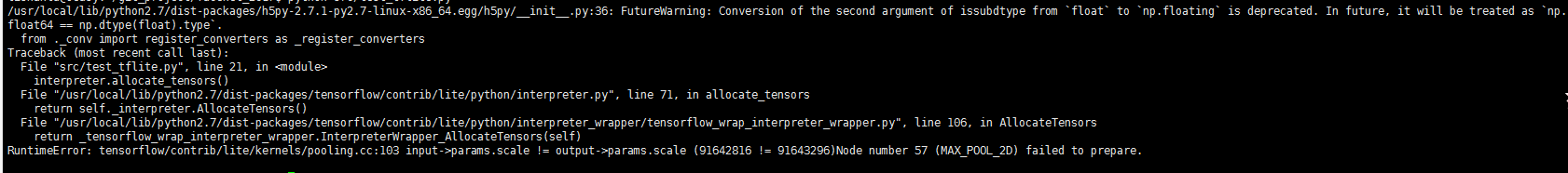出租广告位,需要合作请联系站长

+关注

2020-10(57)

2020-11(18)

## tensorflow实现quantization-aware training（伪量化，fake quantization）

github参考：https://github.com/tensorflow/tensorflow/tree/master/tensorflow/contrib/quantize
tensorflow实例参考：https://github.com/tensorflow/tensorflow/tree/master/tensorflow/examples/speech_commands

# 具体步骤

``````import tensorflow as tf
import os

os.environ["CUDA_VISIBLE_DEVICES"] = "0"
graph_def_file = "train_results/eval_model.pb"
input_arrays = ["input"]
output_arrays = ["softmax"]
converter = tf.contrib.lite.TFLiteConverter.from_frozen_graph(graph_def_file, input_arrays, output_arrays)
converter.inference_type = tf.contrib.lite.constants.QUANTIZED_UINT8
converter.quantized_input_stats = {input_arrays: (73.0, 10.00667)}    # mean, std_dev，需要自己从训练集（增强后，输入网络之前的）统计出来
tflite_model = converter.convert()
open("train_results/freeze_models/converted_model.tflite", "wb").write(tflite_model)
```123456789101112`````````import numpy as np
import tensorflow as tf
import scipy
import os
import cv2

os.environ["CUDA_VISIBLE_DEVICES"] = "0"

# Load TFLite model and allocate tensors.
interpreter = tf.contrib.lite.Interpreter(model_path="train_results/freeze_models/converted_model.tflite")
interpreter.allocate_tensors()

# Get input and output tensors.
input_details = interpreter.get_input_details()
output_details = interpreter.get_output_details()
print(input_details)
print(output_details)

image_q = (image_origin + 7.288) * 10.006671114      #这些参数通过打印input_details可以看到
image_ = np.array([image_q.astype('uint8')])

print(image_.shape)
print(type(image_))

interpreter.set_tensor(input_details['index'], image_)
interpreter.invoke()
output_data = interpreter.get_tensor(output_details['index'])
print(output_data)
print(output_data.shape, type(output_data))

def f(x):
return 0.0078125*(x-128)        # 这些参数也是在output_details里可以看到

output_data_new = map(f, output_data)    #转换后output_data_new为浮点数，你可以和没量化的模型输出对比一下相似度
```1234567891011121314151617181920212223242526272829303132333435```

### 写在后面的话``````image_max = tf.reduce_max(image_batch, name='image_max')
image_min = tf.reduce_min(image_batch, name='image_min')
```12```

tensorflowLite的量化使用问题，帖子很长，慢慢看吧

1 0

pdf(new) 更多>